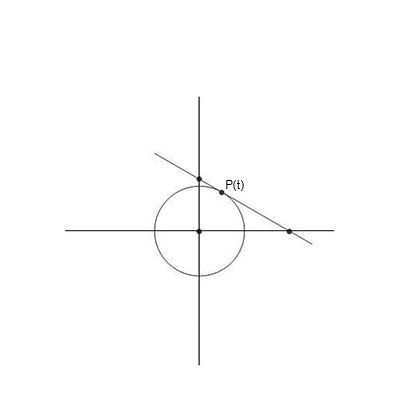# A laser is attached to the unit circle and located at position P(t)=(cos(t),sin(t)) at time t;...

## Question:

A laser is attached to the unit circle and located at position {eq}P(t)=(cos \ t,sin \ t) {/eq} at time {eq}t {/eq}; assume {eq}0 < t < \frac {\pi}{2} {/eq}. The laser points in the direction indicated and hits the {eq}x {/eq}-axis at the position labeled {eq}x(t) {/eq}. Assume time units are seconds and use feet units on the axes of the plane.

a. Determine the area of the right triangle, .

b. {eq}\lim\limits_{ t \to \pi/2} a(t) {/eq}

c. {eq}\lim\limits_{ t \to 0^+} a(t) {/eq}

d. {eq}\lim\limits_{ t \to \pi/4} a(t) {/eq}

e. With restriction on {eq}t {/eq}, what is the smallest {eq}t {/eq} so that {eq}a(t)=2 {/eq}?

f. With restriction on {eq}t {/eq}, what is largest {eq}t {/eq} so that {eq}a(t)=2 {/eq}?

g. What is the average rate of change of the area of the triangle on the time interval {eq}[\pi/6,\pi/4] {/eq}?## Limits and Derivative

This problem is purely analytical and is based on the concept of limits and derivatives. We are going to need to use the concept of derivatives to calculate the rate of change or for the slope followed by the application of the concept of limits to evaluate the given limits. We also need to be familiar with the concept of the average rate of change.

The laser is moving around the unit circle with parametric equations:

{eq}\displaystyle x(t)=\cos t, y(t)=\sin t {/eq}

Location at time t is {eq}\displaystyle P(t)=(\cos t, \sin t) {/eq}

At time t the tangent line to the unit circle at the position {eq}\displaystyle P(t) {/eq} will determine the right angled triangle.

Part A:

We have to calculate the area of the right triangle.

We have,

{eq}\displaystyle x(t)=\cos t, y(t)=\sin t {/eq}

So,

{eq}\displaystyle \frac{dx}{dt}=-\sin t {/eq} and {eq}\displaystyle \frac{dy}{dt}=\cos t {/eq}

Thus, the slope of the tangent is,

{eq}\displaystyle \frac{dy}{dx}=\frac{\frac{dy}{dt}}{\frac{dx}{dt}}=\frac{\cos t}{-\sin t} {/eq}

So, the equation of the tangent is given by,

{eq}\displaystyle y-\sin t =\frac{\cos t}{-\sin t}(x-\cos t) {/eq}

{eq}\displaystyle y\sin t-\sin^2 t =-x\cos t+cos^2 t {/eq}

On simplification, we get,

{eq}\displaystyle \frac{y}{\csc t}+\frac{x}{\sec t}=1 {/eq}

So, the two coordinates of the points where the slope crosses are:

{eq}\displaystyle (\sec t,0) \text{ and }(0, \csc t) {/eq}

Hence, the area of triangle is:

{eq}\displaystyle A=\frac{1}{2}bh {/eq}

{eq}\displaystyle A=\frac{1}{2}\csc t\times \sec t {/eq}

We get, the area of triangle as:

{eq}\displaystyle \boxed{\displaystyle A=a(t)=\frac{1}{\sin 2t}} {/eq}

Part B:

Now, we have to determine,

{eq}\displaystyle \lim_{t\rightarrow \frac{\pi}{2}}a(t) {/eq}

• Here, we need to check the limits from both the sides, as it could be different based on the given information.

{eq}\displaystyle \lim_{t\rightarrow \frac{\pi}{2}+}\left ( \frac{1}{\sin 2t} \right ) {/eq}

Thus, the limit is:

{eq}\displaystyle=-\infty \: {/eq}

And,

{eq}\displaystyle \lim_{t\rightarrow \frac{\pi}{2}-}\left ( \frac{1}{\sin 2t} \right ) {/eq}

{eq}\displaystyle=\infty \: {/eq}

• Therefore, the function diverges at the given point.

Part C:

We have to determine

{eq}\displaystyle L= \lim_{t\rightarrow 0^{+}}\left ( \frac{1}{\sin 2t} \right ) {/eq}

{eq}\displaystyle L= \lim_{h\rightarrow 0}\left ( \frac{1}{\sin 2(0+h)} \right ) {/eq}

{eq}\displaystyle L= \lim_{2h\rightarrow 0}\left ( \frac{1}{\sin 2(h)} \right ) {/eq}

Thus, the required limit is:

{eq}\displaystyle \boxed{\displaystyle L= \left ( \frac{1}{0} \right )=\infty} {/eq}

Part D:

Now, we have to determine

{eq}\displaystyle \lim_{t\rightarrow \frac{\pi}{4}}\left ( \frac{1}{\sin 2t} \right ) {/eq}

So,

{eq}\displaystyle K=\lim_{t\rightarrow \frac{\pi}{4}}\left ( \frac{1}{\sin 2\left ( \frac{\pi}{4} \right )} \right ) {/eq}

{eq}\displaystyle K=\lim_{t\rightarrow \frac{\pi}{4}}\left ( \frac{1}{\sin \left ( \frac{\pi}{2} \right )} \right ) {/eq}

Hence, it is:

{eq}\displaystyle \boxed{\displaystyle K=\left ( \frac{1}{1} \right )=1} {/eq}

Part E:

Now, our aim is to find the value of the smallest {eq}\displaystyle t {/eq} which satisfies {eq}\displaystyle a(t)=2 {/eq}

So,

{eq}\displaystyle \frac{1}{\sin 2t}=2 {/eq}

{eq}\displaystyle \sin 2t=\frac{1}{2} {/eq}

{eq}\displaystyle \sin 2t=\frac{\pi}{6} {/eq}

We can write the general solution as,

{eq}\displaystyle t=\frac{n\pi}{2}+(-1)^n\left ( \frac{\pi}{12} \right ) {/eq}

So for, {eq}\displaystyle n=0 {/eq}

Thus, the smallest value of {eq}\displaystyle t {/eq} such that {eq}\displaystyle a(t)=2 {/eq} is:

{eq}\displaystyle \boxed{t_0=\frac{\pi}{12}} {/eq}

Part F:

Now, we need to find the largest value of {eq}\displaystyle t {/eq} such that {eq}\displaystyle a(t)=2 {/eq}

Let us now find {eq}\displaystyle t_1 {/eq}

{eq}\displaystyle \displaystyle t_1=\frac{1\times \pi}{2}+(-1)^1\left ( \frac{\pi}{12} \right ) {/eq}

{eq}\displaystyle t_1=\frac{\pi}{2}-\left ( \frac{\pi}{12} \right ) {/eq}

{eq}\displaystyle \boxed{\displaystyle t_1=\frac{5\pi}{12}} {/eq}

We have been given that {eq}\displaystyle 0<t<\frac{\pi}{2} {/eq}

• If we consider any other value of {eq}\displaystyle n {/eq} the value of {eq}\displaystyle t {/eq} will be greater than {eq}\displaystyle \frac{\pi}{2} {/eq} or negative.

Thus, the largest value of {eq}\displaystyle t {/eq} is:

{eq}\displaystyle \boxed{t=\frac{5\pi}{12}} {/eq}

Part G:

The average rate of change of area can be given as,

{eq}\displaystyle \text{Avg rate of change of area}=\frac{\frac{1}{\sin \left ( \frac{\pi}{3} \right )}-\frac{1}{\sin\left ( \frac{\pi}{2} \right )}}{\frac{\pi}{4}-\frac{\pi}{6}} {/eq}

{eq}\displaystyle \text{Avg rate of change of area}=\frac{1.15-1}{0.261} {/eq}

Thus, the rate of change of area is:

{eq}\displaystyle \boxed{\displaystyle \text{Avg rate of change of area}=0.574} {/eq}